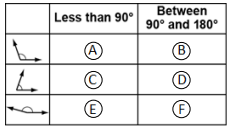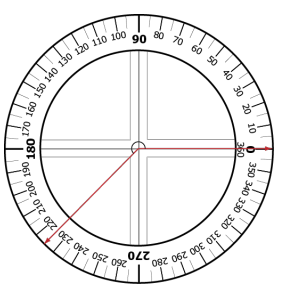# MAFS.4.MD.3.5Archived Standard

Recognize angles as geometric shapes that are formed wherever two rays share a common endpoint, and understand concepts of angle measurement:
1. An angle is measured with reference to a circle with its center at the common endpoint of the rays, by considering the fraction of the circular arc between the points where the two rays intersect the circle. An angle that turns through 1/360 of a circle is called a “one-degree angle,” and can be used to measure angles.
2. An angle that turns through n one-degree angles is said to have an angle measure of n degrees.
General Information
Subject Area: Mathematics
Domain-Subdomain: Measurement and Data
Cluster: Level 1: Recall
Cluster: Geometric measurement: understand concepts of angle and measure angles. (Additional Cluster) -

Clusters should not be sorted from Major to Supporting and then taught in that order. To do so would strip the coherence of the mathematical ideas and miss the opportunity to enhance the major work of the grade with the supporting clusters.

Date Adopted or Revised: 02/14
Content Complexity Rating: Level 1: Recall - More Information
Date of Last Rating: 02/14
Status: State Board Approved - Archived
Assessed: Yes
Test Item Specifications
Also Assesses: MAFS.4.MD.3.6
• Assessment Limits :
Items may contain whole number degree measures within 0° and 360°. For identification, angles are less than 360°. For construction, angles are less than 180°. Items may not require estimating the exact measures of angles.
• Calculator :

No

• Context :

Allowable

Sample Test Items (3)
• Test Item #: Sample Item 1
• Question: Fill in the circles to select the category of measure for each angle.• Difficulty: N/A
• Type: MI: Matching Item

• Test Item #: Sample Item 2
• Question:

An angle is shown.What is the measure, in degrees, of the angle?

• Difficulty: N/A
• Type: EE: Equation Editor

## Related Courses

This benchmark is part of these courses.
5012060: Mathematics - Grade Four (Specifically in versions: 2014 - 2015, 2015 - 2022, 2022 and beyond (current))
7712050: Access Mathematics Grade 4 (Specifically in versions: 2014 - 2015, 2015 - 2018, 2018 - 2022, 2022 and beyond (current))
5020110: STEM Lab Grade 4 (Specifically in versions: 2016 - 2022, 2022 and beyond (current))
5012055: Grade 3 Accelerated Mathematics (Specifically in versions: 2019 - 2022, 2022 and beyond (current))
5012015: Foundational Skills in Mathematics 3-5 (Specifically in versions: 2019 - 2022, 2022 and beyond (current))

## Related Access Points

Alternate version of this benchmark for students with significant cognitive disabilities.

## Related Resources

Vetted resources educators can use to teach the concepts and skills in this benchmark.

## Formative Assessments

This Angle:

Students are given two angles with the same angle measure but with sides of different lengths and asked to explain which component of an angle determines its measure.

Type: Formative Assessment

Lawn Sprinkler:

Students are asked determine angle measures based on how many one-degree turns are made.

Type: Formative Assessment

Determining An Angle’s Measure:

Students are asked to analyze two angles and explain how their measures are determined.

Type: Formative Assessment

Circle the Angles:

Students are asked to circle figures that show angles from a set of figures and then describe the defining attributes of angles.

Type: Formative Assessment

## Image/Photograph

Angles (Clipart ETC):

This large collection of clipart contains images of angles that can be freely used in lesson plans, worksheets, and presentations.

Type: Image/Photograph

## Lesson Plans

Coding Geometry Challenge # 12 & 13:

This set of geometry challenges focuses on creating circles and calculating area/circumference as students problem solve and think as they learn to code using block coding software.  Student will need to use their knowledge of the attributes of polygons and mathematical principals of geometry to accomplish the given challenges. The challenges start out fairly simple and move to more complex situations in which students can explore at their own pace or work as a team. Computer Science standards are seamlessly intertwined with the math standards while providing “Step it up!” and “Jump it up!” opportunities to increase rigor

Type: Lesson Plan

Angles All Around Us:

This lesson will provide students with the opportunity to see angles all around them. Students will be able to see how and where geometry exist in the real world.  Please note that this lesson focuses on identifying acute, obtuse, right, straight and reflex angles.

Type: Lesson Plan

Snowflake Geometry: No Two Alike!:

In this lesson students will make snowflakes, promoting creativity and self-expression, and use them to identify geometric terms. It also gives them an opportunity to follow a sequenced set of instructions for a given outcome.

Type: Lesson Plan

Angles All Around Us:

This is a lesson that introduces right, acute, obtuse, reflex, straight angles in a fun and challenging way.

Type: Lesson Plan

Points, Lines, and Angles, Oh My!:

In this lesson, students work to identify points, lines, line segments, rays, angles and perpendicular and parallel lines. Students create webs of yarn and analyze the web for geometric properties listed above. A teacher can select which vocabulary terms are appropriate for the class.

Type: Lesson Plan

Angle Your Way Around:

In this lesson students will be introduced to the protractor and benchmark angles, practice reading angles properly, make estimates on angle degrees, measure angles precisely, and participate in a small group activity using their new skills.

Type: Lesson Plan

Runway Rotations:

Students will use small paper airplanes to model rotations required to turn onto a runway. Students will rotate planes 45, 90, 180, 270, and 360 degrees. Students will identify and describe the results of rotations using benchmark angles.

Type: Lesson Plan

## Original Student Tutorial

Dash or Bash: Discovering Angles:

Discover what an angle is by helping to program a robot through an obstacle course in this interactive tutorial.

Type: Original Student Tutorial

Finding an unknown angle:

The purpose of this task is to give students a problem involving an unknown quantity that has a clear visual representation. Students must understand that the four interior angles of a rectangle are all right angles and that right angles have a measure of 90° and that angle measure is additive.

## Tutorials

Angles and arcs:

in this tutorial, students will learn about central angles and arcs of a circle.

Type: Tutorial

Recognizing Acute, Right, and Obtuse Angles:

This Khan Academy tutorial video identifies acute, right, and obtuse angles and justifies each identification.

Type: Tutorial

Angle Measurements and Circle Arcs:

This Khan Academy tutorial video demonstrates the relationship between the measurement of an angle and the arc of a circle.

Type: Tutorial

Angle Basics:

This Khan Academy tutorial video presents how an angle is formed and labeled.

Type: Tutorial

## MFAS Formative Assessments

Circle the Angles:

Students are asked to circle figures that show angles from a set of figures and then describe the defining attributes of angles.

Determining An Angle’s Measure:

Students are asked to analyze two angles and explain how their measures are determined.

Lawn Sprinkler:

Students are asked determine angle measures based on how many one-degree turns are made.

This Angle:

Students are given two angles with the same angle measure but with sides of different lengths and asked to explain which component of an angle determines its measure.

## Original Student Tutorials Mathematics - Grades K-5

Dash or Bash: Discovering Angles:

Discover what an angle is by helping to program a robot through an obstacle course in this interactive tutorial.

## Student Resources

Vetted resources students can use to learn the concepts and skills in this benchmark.

## Original Student Tutorial

Dash or Bash: Discovering Angles:

Discover what an angle is by helping to program a robot through an obstacle course in this interactive tutorial.

Type: Original Student Tutorial

Finding an unknown angle:

The purpose of this task is to give students a problem involving an unknown quantity that has a clear visual representation. Students must understand that the four interior angles of a rectangle are all right angles and that right angles have a measure of 90° and that angle measure is additive.

## Tutorials

Angles and arcs:

in this tutorial, students will learn about central angles and arcs of a circle.

Type: Tutorial

Recognizing Acute, Right, and Obtuse Angles:

This Khan Academy tutorial video identifies acute, right, and obtuse angles and justifies each identification.

Type: Tutorial

Angle Measurements and Circle Arcs:

This Khan Academy tutorial video demonstrates the relationship between the measurement of an angle and the arc of a circle.

Type: Tutorial

Angle Basics:

This Khan Academy tutorial video presents how an angle is formed and labeled.

Type: Tutorial

## Parent Resources

Vetted resources caregivers can use to help students learn the concepts and skills in this benchmark.